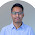## Sunday, 16 September 2012

### Fox in a Hole

Consider 'n' holes in a line. One of them is occupied by a fox. Each night, the fox moves to a neighboring hole, either to the left or to the right. Each morning, you get to inspect a hole of your choice. What strategy would ensure that the fox is eventually caught?

#### 3 comments:

1.does the answer have 2*(n-2) steps?
we go from the second hole to third then next till last but one hole, this sequence would help us catch the fox if he is at an even numbered hole initially
then we start from n-1 and go till 2, this sequence helps us catch the fox if he is at the odd position in the beginning.

2.@admin
jawaab do iss question ka ??

3.The answer by Apoorv is correct
Since, we are starting at hole 2(even hole)
Case 1: Suppose the fox is at even number of hole
After day 1, it would be at odd number hole and we would be also at odd number hole
Similarly, after every day, we both would be wither at even or odd hole
Now, since we are moving from hole 2 to n-1, we would definitely find fox in our way

Case 2: Suppose fox starts at odd hole
We would be never able to find it until we reach n-1 hole
Once we reach n-1 hole, we stay at n-1 hole for a day. This brings us in sync with the fox and thus by moving from n-1 to 2, we can find the fox